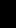Courses

# Test: Torque

## 10 Questions MCQ Test Physics Class 11 | Test: Torque

Description
This mock test of Test: Torque for JEE helps you for every JEE entrance exam. This contains 10 Multiple Choice Questions for JEE Test: Torque (mcq) to study with solutions a complete question bank. The solved questions answers in this Test: Torque quiz give you a good mix of easy questions and tough questions. JEE students definitely take this Test: Torque exercise for a better result in the exam. You can find other Test: Torque extra questions, long questions & short questions for JEE on EduRev as well by searching above.
QUESTION: 1

### A stone of mass m tied to a string of length l is rotating along a circular path with constant speed v. The torque on the stone is

Solution:

Hence the torque is zero .Because the line of action of the tension passes through the centre .So lever arm of tension is zero So the torque acting is also zero .

QUESTION: 2

### An engine develops a power of 360 kw, when rotating at 30 revolutions per second. The Torque required to deliver this power is

Solution:

The power delivered by the torque τ exerted on rotating body is given by
P=τω or τ=P/ω
Here P=360KW=360000 Watt
ω=30 x 2π rad/sec,
ω=60π rad/sec
now,
τ=360000 /60×3.14Nm
τ= 1910.8 Nm

QUESTION: 3

### The dimension ofare

Solution:
QUESTION: 4

Which of the following is an axial vector?

Solution:

Torque is an axial vector. Axial vectors are those vectors which represent rotational effect and act along the axis of rotation. E.g. angular velocity, torque, angular momentum etc. are axial vectors.

QUESTION: 5

Moment of inertia of a solid sphere of mass M and radius R is ______

Solution:
QUESTION: 6

A wheel having moment of inertia 3.5 kgm2 rotates about the vertical axis. The torque required to produce an angular acceleration of 25rad/s2 in the wheel is

Solution:

Torque = moment of inertia × angular acceleration
3.5 × 25 =87.5 Nm

QUESTION: 7

For a system to be in equilibrium, the net torques acting on it must balance. This is true only if the torque are taken about

Solution:

For a system to be in equilibrium torques on the system must be equal to zero
This is true only if the torques are taken about the centre of mass as the whole mass of the object seems to be situated at centre of mass.

QUESTION: 8

The rotational analog to the expression F = ma in linear motion is ___________in rotational motion.

Solution:

Force (in linear) = torque (in rotational)
Mass (in linear motion) = moment of inertia ( l ) (in rotational motion)
Acceleration(a) (in linear) = angular acceleration (α) (in rotational)
F = ma (in linear) and torque = Iα (in rotational)

QUESTION: 9

Rotational analogue of force of linear motion is

Solution:

Rotational analogue of force in linear motion is torque. Torque is the turning effect of force.

QUESTION: 10

When no external Torque acts on a system, what is conserved

Solution:

τ = dI/dt
If τ = 0 then L is conserved.